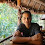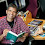## Saturday, April 30, 2011

### Alternatives to the CAPM: Part 3: Connecting cost of debt to cost of equity

Analysts have generally had an easier time estimating the cost of debt than the cost of equity, for any given firm, for a simple reason. When banks lend money to a firm, the cost of debt is explicit at least at the time of borrowing and takes the form of an interest rate. While it is true that this stated interest rate may not be a good measure of cost of debt later in the loan life, the cost of debt for firms with publicly traded bonds outstanding can be computed as the yield to maturity (an observable and updated number) on those bonds.

Armed with this insight, there are some who suggest that the cost of equity for a firm can be estimated, relative to its cost of debt. Their intuition goes as follows. If the pre-tax cost of debt for a firm is 8% its cost of equity should be higher. But how much higher? One approach that has been developed is to estimate the standard deviation in bond and stock returns for a company; both numbers should be available if both instruments are traded. The cost of equity then can be written as follows:
Cost of equity = Cost of debt (Standard deviation of equity/ Standard deviation of bond)
Thus, in the example above, if the standard deviation in stock prices is 30% and the standard deviation in bond prices is only 20%, the cost of equity will be 12%.
Cost of equity = 8% (30/20) = 12%
In fact, an alternative to using historical standard deviations is to use implied standard deviations, assuming that there are options outstanding on the stock, the bond or on both.

While this approach seems appealing, it is both dangerous and has very limited use. Note that it works only for publicly companies that have significant debt outstanding in the form of corporate bonds. Since these firms are generally large market cap companies, with long histories, they also tend to be companies where estimating the cost of capital using conventional approaches is easiest. This approach cannot be used for large market companies like Apple and Google that have no debt outstanding or for any company that has only bank debt (since it is not traded and has no standard deviation). There is also the underlying problem that the risk of investing in equity (where you get residual cash flows, and the uncertainty is about the magnitude of these cash flows) is very different from the risk of investing in the company's bonds (where the risk is that you will not get promised payments - the upside is limited and the downside is high) and the ratio of their standard deviations may be a poor indication of risk, at least for individual companies. It also assumes that all of the risk in equity is relevant, even though a large portion of that risk may disappear in portfolios. Consequently, you will overstate the cost of equity for firms where the bulk of the risk is firm-specific and not market risk.

Notwithstanding these limitations, this approach can still be used as a check on costs of equity estimated using other approaches, especially for companies that have significant debt outstanding. Since the claims of equity investors can be met only after lenders' claims have been met, it is logical that the cost of equity should be higher than the pre-tax cost of debt, with the difference increasing with the proportion of cash flows being used to service debt payments. Using a simple proxy for this proportion - interest coverage ratio (operating income/ interest expense), for instance, I would hypothesize that the cost of equity will rise, relative to the cost of debt, as the interest coverage ratio decreases. Incidentally, this is the same rationale that we use to adjust betas for financial leverage, with beta increasing as the debt to equity ratio increases.

The series on alternatives to the CAPM
Alternatives to the CAPM: Part 1: Relative Risk Measures
Alternatives to the CAPM: Part 2: Proxy Models
Alternatives to the CAPM: Part 3: Connecting cost of equity to cost of debt
Alternatives to the CAPM: Part 4: Market-implied costs of equity
Alternatives to the CAPM: Part 5: Risk adjusting the cash flows
Alternatives to the CAPM: Wrapping up

1.Dear Prof,

Thanks for the enlightening series! Also wanted to understnad a bit about intutively calculating the cost of equity for a firm relative to its cost of debt. The estimation assumes that there is some kind of relation bettween the CoVs of debt and equity of a company.
CoV would be higher for equity most of the times since it gets the residual cashflows. However some times like during peiod of volatility in debt markets, credit rating change of the company etc. CoV for debt might be higher.
Wouldn't it be advisable to avoid this measure altogether rather than trying to find relation between debt and equity CoVs of a firm? Or would it have some advantages like ensuring that companies with lower cost of debt have lower cost of equity too for an industry?

2.If the debt market is volatile, the equity market will be even more so, and if there is a ratings change, it is because of fundamental shifts in the company which should show up in the cost of equity.

3.Professor,

Appreciate your valuable insights into this issue!

How can we directly compare the risks of debt with those of equity where IMHO they are measuring different versions of risk? I.E. debt measures bankruptcy or loss of capital whereas equity measures variability in returns on capital. In my opinion equity investors by default factor in a default premium when they pay a particular price for a stock and doesn't need additional adjustment!

Following on from this how would variances in two variables be directly comparable to each other at all. And if my line of thinking is wrong, then investors shouldn't be assessing absolute level of returns from debt or equity but more concerned with exploiting mis-pricing differentials amongst the two if they share a dependable relationship.

Would value your thoughts into this!

Thanks

4.I would love to find alternative to capm.I prefer a build up method.So from risk free we can go see the bond rating and see a typical yield, if there is no rating you have shown a good way to estimate a rating with the interest coverage ratio. So we have the first part Cost of equity should be greater than risk free+ bond spread. Then we can see the standard deviation of stocks with a given rating compared to the standard deviation of the bonds with the same rating. So we here we have it

Cost of equity= Rf+(bond spread)*(σ stocks with rating x/σbond with rating x )

interesting to see how much this deviates from the Capm model

Given the amount of spam that I seem to be attracting, I have turned on comment moderation. I have to okay your comment for it to appear. I apologize for this intermediate oversight, but the legitimate comments are being drowned out by the sales pitches and spam.